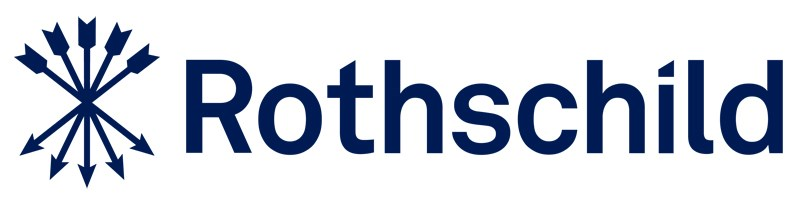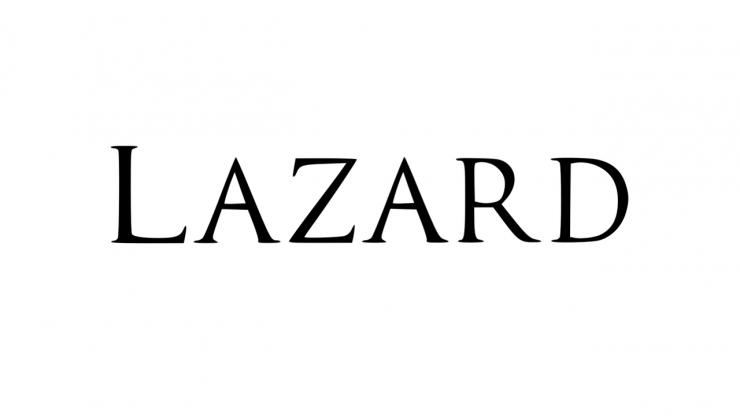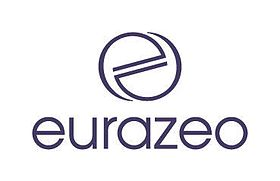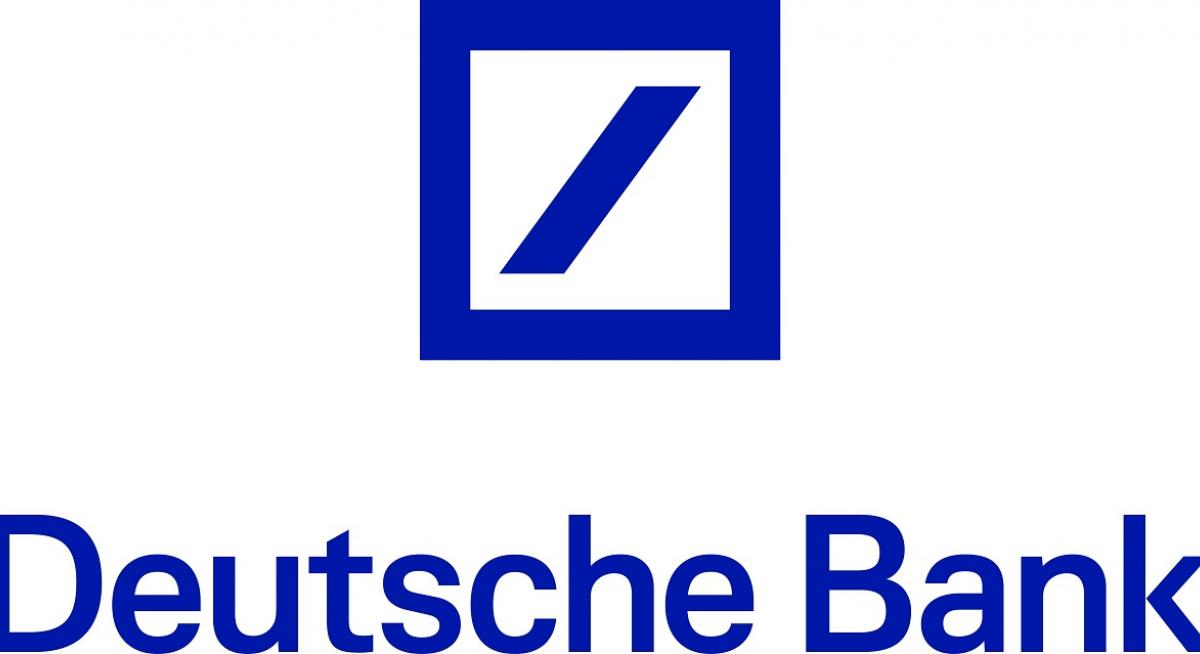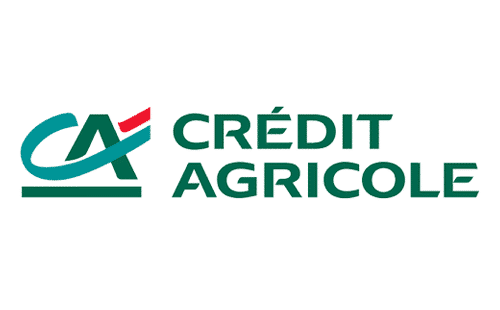# Course curriculum

• 1

### Overview

• What are the principal valuation methods?
• 2

### Relative valuation

• What are the principal steps to take in relative valuation?
• What criteria would you use to select comparables?
• What multiples would you choose to use and why?
• What are the steps involved in calculating trading multiples?
• What are the steps involved in calculating precedent transactions?
• 3

### Intrinsic valuation - DCF

• What are the steps involved in performing a DCF analysis? - Part 1
• What are the steps involved in performing a DCF analysis? - Part 2
• What are the steps involved in performing a DCF analysis? - Part 3
• How do we calculate Free Cash Flow in a DCF?
• How do we calculate cost of capital?
• How do we calculate the cost of equity?
• What is beta?
• Concretely, how might we derive the beta of a particular company?
• How do we calculate the terminal value of a company?
• Which valuation method results in the highest valuation?
• Which of the 3 valuation methods should you use as a matter of priority?

# Our students have secured offers in some of the most prestigious companies in M&A and private equity